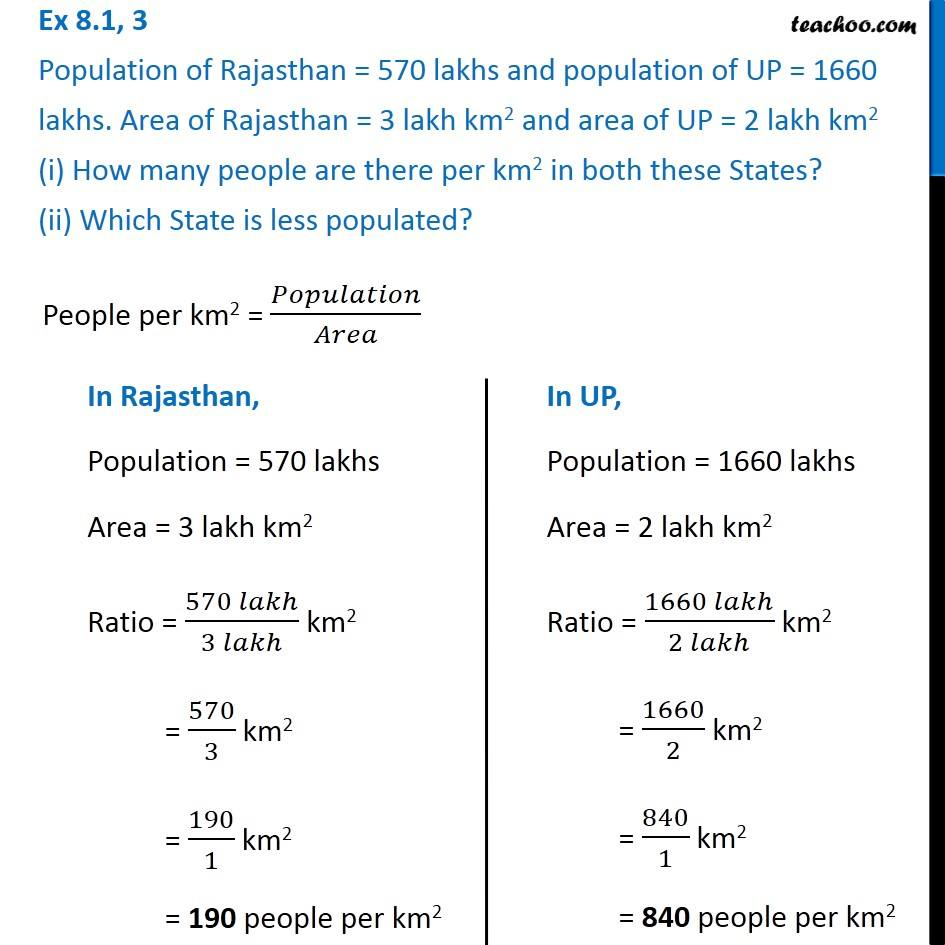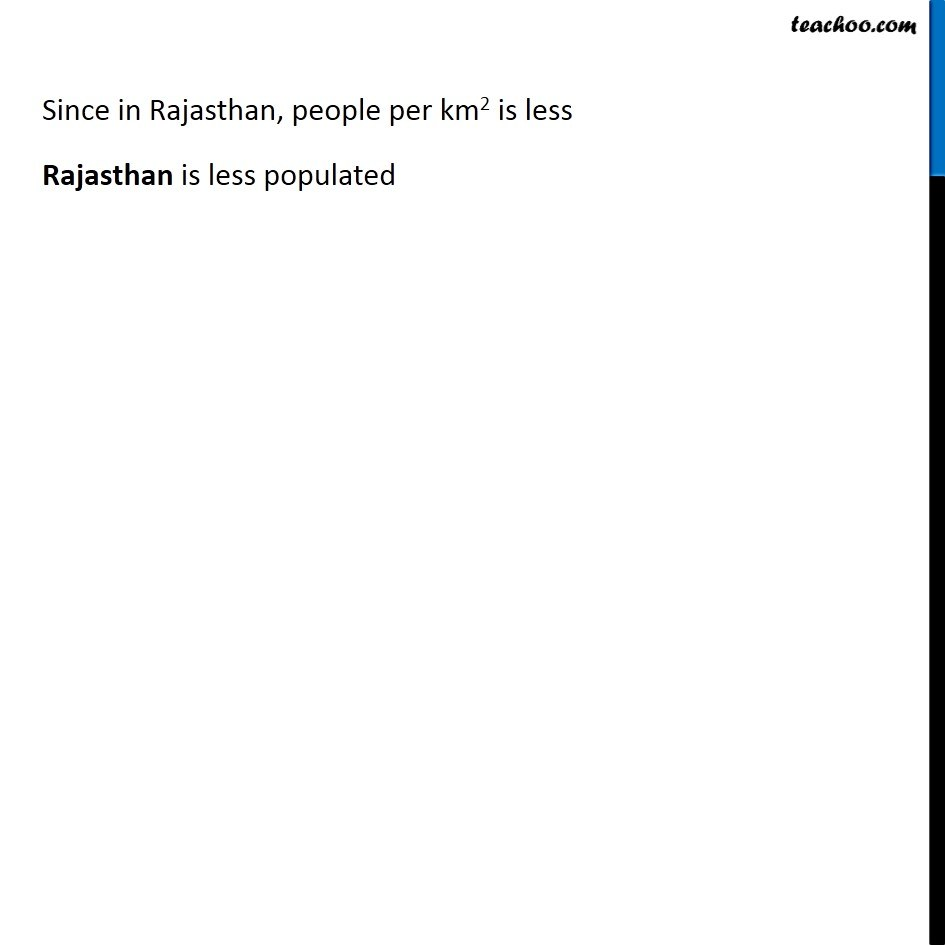Finding Ratios

Chapter 7 Class 7 Comparing Quantities
Serial order wiseLearn in your speed, with individual attention - Teachoo Maths 1-on-1 Class

### Transcript

Question 3 Population of Rajasthan = 570 lakhs and population of UP = 1660 lakhs. Area of Rajasthan = 3 lakh km2 and area of UP = 2 lakh km2 (i) How many people are there per km2 in both these States? (ii) Which State is less populated? People per km2 = 𝑃𝑜𝑝𝑢𝑙𝑎𝑡𝑖𝑜𝑛/𝐴𝑟𝑒𝑎 In Rajasthan, Population = 570 lakhs Area = 3 lakh km2 Ratio = (570 𝑙𝑎𝑘ℎ)/(3 𝑙𝑎𝑘ℎ) km2 = 570/3 km2 = 190/1 km2 = 190 people per km2 In UP, Population = 1660 lakhs Area = 2 lakh km2 Ratio = (1660 𝑙𝑎𝑘ℎ)/(2 𝑙𝑎𝑘ℎ) km2 = 1660/2 km2 = 840/1 km2 = 840 people per km2 Since in Rajasthan, people per km2 is less Rajasthan is less populated